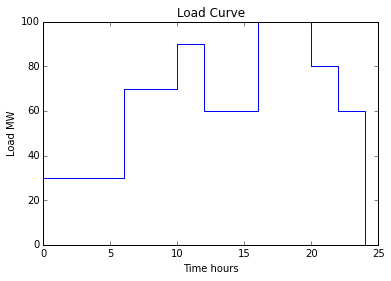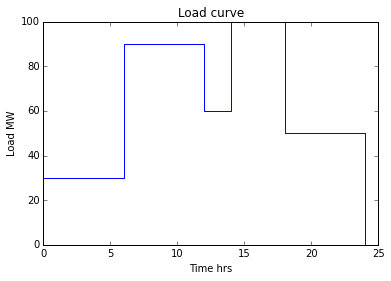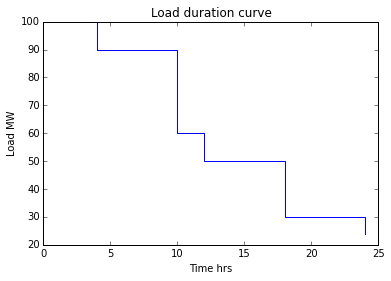# Chapter 1 : Introduction : Economics of Power Generation¶

## Ex1.1 Page23¶

In :
from __future__ import division
%matplotlib inline
from matplotlib.pyplot import plot, title, xlabel, ylabel, show
#Input data
C=30#Capacity in MW
M=70#Loads are taken above 70 MW
t1=[0,6]#Time range in hours
t2=[6,10]#Time range in hours
t3=[10,12]#Time range in hours
t4=[12,16]#Time range in hours
t5=[16,20]#Time range in hours
t6=[20,22]#Time range in hours
t7=[22,24]#Time range in hours

#Calculations
E=((L*(t1-t1))+(L*(t2-t2))+(L*(t3-t3))+(L*(t4-t4))+(L*(t5-t5))+(L*(t6-t6))+(L*(t7-t7)))#Energy generated in MWh
E1=((L-M)*(t3-t3))+((L-M)*(t5-t5))+((L-M)*(t6-t6))#Energy generated if the load above 70 MW is supplied by a standby unit of 30 MW capacity in MWh
T=(t3-t3)+(t5-t5)+(t6-t6)#Time during which the standby unit remains in operation in h
U=(E1/(C*T))#Use factor

#Output
t=[0,0,6,6,10,10,12,12,16,16,20,20,22,22,24,24]#Time for plotting load curve in hours
plot(t,l)#Load curve taking Time in hours on X-axis and Load in MW on Y- axis
xlabel('Time hours')
print "(a)Load factor of the plant is %3.2f\n(b)Load factor of a standby equipment of %3.0f capacity if it takes up all the loads above %3.0f MW is %3.2f\n(c)Use factor is %3.2f"%(LF,C,M,LF1,U)

(a)Load factor of the plant is 0.65
(b)Load factor of a standby equipment of  30 capacity if it takes up all the loads above  70 MW is 0.75
(c)Use factor is 0.75## Ex1.2 Page25¶

In :
from __future__ import division
#Input data
P=60#Peak load on power plant in MW
L=[30,20,10,14]#Loads having maximum demands in MW
C=80#Capacity of the power plant in MW
Y=8760#Number of hours in a year of 365 days

#Calculations
E=(AL*1000*Y)/10**6#Energy supplied per year in kWh*10**6
DF=(P/(L+L+L+L))#Demand factor
DIF=((L+L+L+L)/P)#Diversity factor

#Output
print "(a) The average load on the power plant is %3.0f MW \n(b) The energy supplied per year is %3.1f *10**6 kWh \n(c) Demand factor is %3.3f \n(d) Diversity factor is %3.3f"%(AL,E,DF,DIF)

(a) The average load on the power plant is  30 MW
(b) The energy supplied per year is 262.8 *10**6 kWh
(c) Demand factor is 0.811
(d) Diversity factor is 1.233


## Ex1.3 Page25¶

In :
#Input data
C=210#Capacity of thermal power plant in MW
m=1#Coal consumption per kWh of energy generated
Rs=450#Cost of coal in Rs per tonne
Y=8760#Number of hours in a year of 365 days

#Calculations
E=(AL*Y)#Energy generated per year in MWh
CL=(E*1000)#Coal required per year in kg
CY=(E*Rs)#Cost of coal per year
CE=CL#Cost of energy sold in Rs
RY=(CE-CY)/10**7#Revenue earned by the power plant per year in Rs crore
CF=(AL/C)#Capacity factor

#Output
print "(a) The annual revenue earned by the power plant is Rs %3.2f crore \n(b) Capacity factor is %3.3f"%(RY,CF)

(a) The annual revenue earned by the power plant is Rs 46.25 crore
(b) Capacity factor is 0.457


## Ex1.4 Page 26¶

In :
#Input data
C=0.60#Capacity factor
U=0.65#Use factor
M=60#Maximum power demand in MW
Y=8760#Number of hours in a year of 365 days

#Calculations
P=((A*1000)*Y)/10**6#Annual energy production in kWh *10**6
PC=(A/C)#Plant capacity in MW
R=(PC-M)#Reserve capacity in MW
HIO=(P*1000/(U*PC))#Hours in operation in hrs
NH=(Y-HIO)#Hours not in service in a year in hrs

#Output
print "(a) Annual energy production is %3.1f * 10**6 kWh \n(b) Reserve capacity over and above the peak load is %3.0f MW \n(c) The hours during which the plant is not in service per year is %3.0f hrs"%(P,R,NH)

(a) Annual energy production is 394.2 * 10**6 kWh
(b) Reserve capacity over and above the peak load is  15 MW
(c) The hours during which the plant is not in service per year is 674 hrs


## Ex1.5 Page26¶

In :
from __future__ import division
#Input data
Dd=500#Maximum demand in MW
#1)Steam power plant 2)Hydroelectric power plant 3)Nuclear power plant
CC=[0,3,4,5]#Capital cost per MW installed in Rs. crore
I=[0,6,5,5]#Interest in percent
D=[0,6,4,5]#Depreciation in percent
OP=[0,30,5,15]#Operating cost (including fuel) per kWh
TD=[0,2,3,2]#Transmission and distribution cost per kWh
Y=8760#Number of hours in a year of 365 days

#Calculations
#1)Steam power plant
CCX=(CC[(1)]*Dd*10**7)#Capital cost in Rs
IX=((I[(1)]/100)*CCX)#Interest in Rs
DX=((D[(1)]/100)*CCX)#Depreciation in Rs
AFCX=IX+DX#Annual fixed cost in Rs
EX=(L*Dd*1000*Y)#Energy generated per year in kWh
RX=(OP[(1)]+TD[(1)])#Running cost/kWh in paise
OX=((AFCX/EX)+(RX/100))*100#Overall cost/kWh in paise

#2)Hydroelectric Power plant
CCY=(CC[(2)]*Dd*10**7)#Capital cost in Rs
IY=((I[(2)]/100)*CCY)#Interest in Rs
DY=((D[(2)]/100)*CCY)#Depreciation in Rs
AFCY=IY+DY#Annual fixed cost in Rs
EY=(L*Dd*1000*Y)#Energy generated per year in kWh
RY=(OP[(2)]+TD[(2)])#Running cost/kWh in paise
OY=((AFCY/EY)+(RY/100))*100#Overall cost/kWh in paise

#3)Nuclear power plant
CCZ=(CC[(3)]*Dd*10**7)#Capital cost in Rs
IZ=((I[(3)]/100)*CCZ)#Interest in Rs
DZ=((D[(3)]/100)*CCZ)#Depreciation in Rs
AFCZ=IZ+DZ#Annual fixed cost in Rs
EZ=(L*Dd*1000*Y)#Energy generated per year in kWh
RZ=(OP[(3)]+TD[(3)])#Running cost/kWh in paise
OZ=((AFCZ/EZ)+(RZ/100))*100#Overall cost/kWh in paise

#Output
print "(i)Overall cost per kWh in Steam power plant is %3.0f paise \n(ii)Overall cost per kWh in Hydroelectric power plant is %3.0f paise \n(iii)Overall cost per kWh in Nuclear power plant is %3.0f paise"%(OX,OY,OZ)

(i)Overall cost per kWh in Steam power plant is  91 paise
(ii)Overall cost per kWh in Hydroelectric power plant is  67 paise
(iii)Overall cost per kWh in Nuclear power plant is  99 paise


## Ex1.6 Page28¶

In :
from __future__ import division
#Input data
C=210#Capacity in MW
ID=12#Interest and depreciation in percent
CC=18000#Capital cost/kW installed in Rs
AC=0.54#Annual capacity factor
RC=(200*10**6)#Annual running charges in Rs
E=6#Energy consumed by power plant auxiliaries in percent
Y=8760#Number of hours in a year of 365 days

#Calculations
MD=(C/L)*AC#Maximum demand in MW
RSC=(C-MD)#Reserve Capacity in MW
EP=(AL*1000*Y)#Energy produced per year in kWh
NE=((100-E)/100)*EP#Net energy delivered in kWh
AID=((ID/100)*CC*C*1000)#Annual interest and depreciation in Rs
T=(AID+RC)#Total annual cost in Rs
CP=(T/NE)*100#Cost of power generation in paise

#Output
print "(a) The cost of power generation per kWh is %3.0f paise \n(b) The reserve capacity is %3.0f MW"%(CP,RSC)

(a) The cost of power generation per kWh is  70 paise
(b) The reserve capacity is  21 MW


## Ex1.7 Page28¶

In :
from numpy import mat
#Input data
L=200#The total load supplied by the plants in MW
#The incremental fuel costs for generating units a and b of power plant are given by
#dFa/dPa=0.065Pa+25
#dFb/dPb=0.08Pa+20

#Calculations
#Solving two equations
#Pa+Pb=200
#0.065Pa+25=0.08Pb+20
A=mat([[1, 1],[0.065, -0.08]])#Coefficient matrix
B=mat([[L],[(20-25)]])#Constant matrix
X=(A**-1)*B#Variable matrix
P=100#If load is shared equally then Pa=Pb=100MW
a=(((0.065*P**2)/2)+(25*P))-(((0.065*X**2)/2)+(25*X))#increase in fuel cost for unit a in Rs. per hour
b=(((0.08*P**2)/2)+(20*P))-(((0.08*X**2)/2)+(20*X))#increase in fuel cost for unit a in Rs. per hour
x=a+b#Net increase in fuel cost due to departure from economic distribution of load in Rs. per hour

#Output
print "(a)The economic loading of two units when the total load supplied by the power plants is 200 MW are %3.2f MW and %3.2f MW\n(b)The loss in fuel cost per hour if the load is equally shared by both units is Rs.%3.2f per hour"%(X,X,x)

(a)The economic loading of two units when the total load supplied by the power plants is 200 MW are 75.86 MW and 124.14 MW
(b)The loss in fuel cost per hour if the load is equally shared by both units is Rs.42.24 per hour


## Ex1.8 page29¶

In :
from math import ceil
#Input data
C=200#Installed capacity of the plant in MW
CC=400#Capital cost in Rs crores
ID=12#Rate of interest and depreciation in percent
AC=5#Annual cost of fuel, salaries and taxation in Rs. crores
Y=8760#Number of hours in a year of 365 days

#Calculations
E=(AvL*1000*Y)#Energy generated per year in kWh
IDC=((ID/100)*CC*10**7)#Interest and depreciation (fixed cost) in Rs
T=(IDC+(AC*10**7))#Total annual cost in Rs
CP1=(T/E)*100#Cost per kWh in paise
E2=(AvL2*1000*Y)#Energy generated per year in kWh
CP2=(T/E2)*100#Cost per kWh in paise
S=((CP1)-(CP2))#Saving in cost per kWh in paise
S1=ceil(S)#Rounding off to next higher integer

#Output
print " Cost of generation per kWh is %3.0f paise \n Saving in cost per kWh if the annual load factor is raised to 60 percent is %3.0f paise"%(CP1,S1)

 Cost of generation per kWh is  61 paise
Saving in cost per kWh if the annual load factor is raised to 60 percent is  11 paise


## Ex1.9 Page30¶

In :
#Input data
C=300#Capacity of power plant in MW
MXD=240#Maximum demand in MW in a year
MND=180#Minimum demand in MW in a year
#Assuming the load duration curve shown in Figure E1.9 on page no 30 to be straight line
Y=8760#Number of hours in a year of 365 days

#Calculations
E=((MND*Y)+0.5*(MXD-MND)*Y)*1000#Energy supplied per year in kWh
CF=((AL/1000)*Y)/(C*Y)#Capacity factor

#Output
print "(a) Load factor is %3.3f \n(b) Capacity factor is %3.2f"%(L,CF)

(a) Load factor is 0.875
(b) Capacity factor is 0.70


## Ex1.10 Page 31¶

In :
#Input data
C=60#Capacity of power plant in MW
MXD=50#Maximum demand in MW in a year
cc = 1 # kg/unit (Coal consumption)
c_cost = 600 # Rs/Tonne
e_cost = 2 # Rs/kWh
Y=8760#Number of hours in a year of 365 days

#Calculations
E=AL*10**3*Y #Energy generated per year in kWh
Coal = E*cc/10**3 # Tonnes (Coal required per year)
CC = Coal*c_cost # rupees (Coal cost / year)
CE = E*e_cost # Rs (Cost of energy sold)
Rev = CE-CC
#Output
print "Revenue earned by the power plant = %0.3e Rs./year"%(Rev)

Revenue earned by the power plant = 3.679e+08 Rs./year


## Ex1.11 Page31¶

In :
%matplotlib inline
from matplotlib.pyplot import plot,subplot,title,xlabel,ylabel,show
#Input data
t1x=[0,6]#Time range in hours
t2x=[6,12]#Time range in hours
t3=[12,14]#Time range in hours
t4=[14,18]#Time range in hours
t5=[18,24]#Time range in hours

#Calculations
t1=[0,6,6,12,12,14,14,18,18,24,24]#Time in hours for Load curve
t2=[0,4,4,10,10,12,12,18,18,24,24]#Time in hours for Load duration curve
E=((L*(t1x-t1x))+(L*(t2x-t2x))+(L*(t3-t3))+(L*(t4-t4))+(L*(t5-t5)))#Energy generated in MWh
MD=max(L,L,L,L,L)#Maximum demand in MW
Lx=[30,10]#Loads for selecting suitable generating units in MW
tx=[24,18,10,4]#Time for selecting suitable generating units in hrs
PC=(Lx*tx+Lx*1)#Plant capacity in MW
CF=(E/(PC*24))#Capacity factor

#Output
plot(t1,L1)#Load curve taking Time in hrs on X- axis and Load in MW on Y- axis
xlabel('Time hrs')
show()
plot(t2,L2)#Load duration curve taking Time in hrs on X- axis and Load in MW on Y- axis
xlabel('Time hrs')
show()
print "(c)Suitable generating units to supply the load are\ni)One unit of %3.0f MW will run for %3.0f hours\nii)One unit of %3.0f MW will run for %3.0f hours\niii)One unit of %3.0f MW will run for %3.0f hours\niv)One unit of %3.0f MW will run for %3.0f hours\n\n(d)Load factor is %3.2f\n\n(e)Capacity of the plant is %3.0f MW and Capacity factor is %3.3f"%(Lx,tx,Lx,tx,Lx,tx,Lx,tx,LF,PC,CF)(c)Suitable generating units to supply the load are
i)One unit of  30 MW will run for  24 hours
ii)One unit of  30 MW will run for  18 hours
iii)One unit of  30 MW will run for  10 hours
iv)One unit of  10 MW will run for   4 hours

(e)Capacity of the plant is 130 MW and Capacity factor is 0.494


## Ex1.12 Page32¶

In :
#Input data
C=10#Capacity of generating unit in MW
MD=[6,3.6,0.4]#Maximum demand for domestic consumers, industrial consumers and street-lighting load respectively in MW
CC=10000#Capital cost of the plant per kW in Rs
RC=3600000#Total rumming cost per year in Rs
AID=10#Annual interest and depreciation on capital cost in percent
Y=8760#Number of hours in a year of 365 days

#Calculations
E=((MD*L)+(MD*L)+(MD*L))*Y*1000#Energy supplied per year to all three consumers in kWh
OC=(RC/E)#Operating charges per kWh in Rs
CCP=(C*1000*CC)#capital cost of the plant in Rs
FCY=((AID/100)*CCP)#Fixed charges per year in Rs
FCkW=(FCY/CC)#Fixed charges per kW in Rs
#a) For domestic consumers
TC1=((FCkW*MD*1000)+(OC*MD*L*Y*1000))#Total chrges in Rs
OC1=(TC1/(MD*L*Y*1000))*100#Overall cost per kWh in paise
#b)For industrial consumers
TC2=((FCkW*MD*1000)+(OC*MD)*L*Y*1000)#Total chrges in Rs
OC2=(TC2/(MD*L*Y*1000))*100#Overall cost per kWh in paise
TC3=((FCkW*MD*1000)+(OC*MD)*L*Y*1000)#Total chrges in Rs
OC3=(TC3/(MD*L*Y*1000))*100#Overall cost per kWh in paise

#Output
print "Overall cost of energy per kWh for:\n(a)Domestic consumers is %3.0f paise\n(b)Industrial consumers is %3.0f paise\n(c)Street-lighting load is %3.0f paise"%(OC1,OC2,OC3)

Overall cost of energy per kWh for:
(a)Domestic consumers is  70 paise
(b)Industrial consumers is  36 paise


## Ex1.13 Page32¶

In :
#Input data
CC=(80*10**6)#Capital cost in Rs
L=30#Useful life in years
S=5#Salvage value of the capital cost in percent
i=0.06#Yearly rate of compound interest

#Calculations
A=((100-S)/100)*CC#Difference of capital cost and salvage value in Rs
P=((A*i)/((1+i)**L-1))#The amount of money to be saved annually in Rs

#Output
print "The amount of money to be saved annually is Rs.%3.0f/-"%(P)

The amount of money to be saved annually is Rs.961317/-


## Ex1.14 Page34¶

In :
#Input data
i=4000#Initial investment in Rs crore
Y=4#Period in years
B=400#Amount paid from 5th year onwards to the 12th year in Rs crore
a=5#5th year
b=12#12th year
y=30#Period in years
C=600#Salvage value in Rs crore
I=0.1#Interest rate

#Calculations
X=(1/(1+I))#X value for calculations
PW=(i+(A*X**Y)+((B/I)*X**b*((I+1)**b-1))-((B/I)*X**a*((I+1)**a-1))-(C*X**y))#Present worth of the payments at the time of commissioning in Rs. crores

#Output
print "Present worth of the payments at the time of commissioning is Rs.%3.2f crores"%(PW)

Present worth of the payments at the time of commissioning is Rs.5994.39 crores


## Ex1.15 Page 35¶

In :
#Input data
O=1000#Combined output of two units in MW
#Two coal generating units P and Q have the incremental heat rate defined by
#(IR)P=0.4818*10**-7.LP**4 - 0.9089*10**-4.LP**3 + 0.6842*10**-1.LP**2 - 0.2106*10.LP + 9860
#(IR)R=0.9592*10**-7.LQ**4 - 0.7811*10**-4.LQ**3 + 0.2625*10**-1.LQ**2 - 0.2189*10.LQ + 9003

#Calculations
#LP+LQ=1000
#By making (IR)P=(IR)Q and solving the above three equations by a numerical methos such as Newton-Raphson algorithm, we get
LP=732.5#Heat rate in MW
LQ=(O-LP)#Heat rate in MW
IR=0.4818*10**-7*LP**4 - 0.9089*10**-4*LP**3 + 0.6842*10**-1*LP**2 - 0.2106*100*LP + 9860
IR1=0.9592*10**-7*LQ**4 - 0.7811*10**-4*LQ**3 + 0.2625*10**-1*LQ**2 - 0.2189*10*LQ + 9003

#Output
print "Incremental heat transfer rate at which the combined output of the two units is %3.0f MW is IR = (IR)P = (IR)Q = %d kJ/kWh"%(O,IR)

Incremental heat transfer rate at which the combined output of the two units is 1000 MW is IR = (IR)P = (IR)Q = 9293 kJ/kWh


## Ex1.16 Page 36¶

In :
#Input data
F=2700#Fixed cost of the thermal station per kW of installed capacity per year in Rs,
FO=40#Fuel and operating costs per kWh generated in paise
Y=8760#Number of hours in a year of 365 days

#Calculations
FC=(F/Y)*100#Fixed costs per kW per hour in paise
E1=(L/100)#Energy produced in 1 hr with 1 kW plant in kWh
FOC1=(E1*FO)#Fuel and operating cost in paise
TC1=(FC+FOC1)#Total cost per hr in paise
C1=(TC1/E1)#Cost per kWh in paise
E2=(L/100)#Energy produced in 1 hr with 1 kW plant in kWh
FOC2=(E2*FO)#Fuel and operating cost in paise
TC2=(FC+FOC2)#Total cost per hr in paise
C2=(TC2/E2)#Cost per kWh in paise
E3=(L/100)#Energy produced in 1 hr with 1 kW plant in kWh
FOC3=(E3*FO)#Fuel and operating cost in paise
TC3=(FC+FOC3)#Total cost per hr in paise
C3=(TC3/E3)#Cost per kWh in paise
E4=(L/100)#Energy produced in 1 hr with 1 kW plant in kWh
FOC4=(E4*FO)#Fuel and operating cost in paise
TC4=(FC+FOC4)#Total cost per hr in paise
C4=(TC4/E4)#Cost per kWh in paise

#Output
print "==============================================================================\nLoad      Energy produced    Fixed cost   Fuel and       Total cost     Cost per\nfactor    in 1hr with        per hr      operating cost    per hr        kWh\n(percent) 1kW plant(kWh)    (paise)        (paise)        (paise)        (paise)\n==============================================================================\n%3.0f          %3.0f               %3.0f            %3.0f            %3.0f          %3.0f\n%3.0f          %3.2f              %3.0f            %3.0f            %3.0f          %3.0f\n%3.0f          %3.2f              %3.0f            %3.0f            %3.0f          %3.0f\n%3.0f          %3.2f              %3.0f            %3.0f            %3.0f          %3.0f\n=============================================================================="%(L,E1,FC,FOC1,TC1,C1,L,E2,FC,FOC2,TC2,C2,L,E3,FC,FOC3,TC3,C3,L,E4,FC,FOC4,TC4,C4)

==============================================================================
Load      Energy produced    Fixed cost   Fuel and       Total cost     Cost per
factor    in 1hr with        per hr      operating cost    per hr        kWh
(percent) 1kW plant(kWh)    (paise)        (paise)        (paise)        (paise)
==============================================================================
100            1                31             40             71           71
75          0.75               31             30             61           81
50          0.50               31             20             51          102
25          0.25               31             10             41          163
==============================================================================